Next: Algorithm Up: Compton Process Previous: Formulas

### Usage

First you must initialize the event generators, i.e., create a table of the functions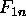and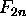. They are three-argument functions. In the program,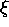,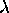, and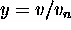(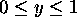) are used as the independent variables. To initialize the generators, you must specify the range and number of mesh points for these variables.

The present version allows to enhance the probability of a part of spectrum. See below.

1ex

C ALL NLCPST(MY,MPH,MXI,MLM,XIMAX,LMMAX,LENHCP,ENHCPF,IRTN)

MY
Number of y's. (i-th y point is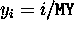,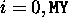)
MPH
Maximum number of laser photons. (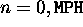)
MXI
Number of's. (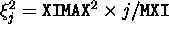,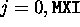)
MLM
Number of's. (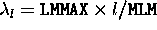,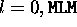)
XIMAX
Maximum.
LMMAX
Maximum.
LENHCP
Flag to apply a rate enhancement function.
ENHCPF
Enhancement function name declared external. Used when LENHCP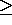1.
IRTN
Return code.
A second call of NLCPST will replace the parameters and the arrays created in the first call.

The enhancement function, if needed, has to be defined as

```            FUNCTION ENHCPF(Y)
REAL*8 ENHCPF,Y
ENHCPF=......
RETURN
END```
The enhancement function has to be a function of() only and it must be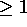for all y. The probability functions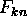are multiplied by ENHCPF(y). Note that y close to 1 represents events with high energy photons and, hence, low energy recoil electrons. See NLCPGN for how the enhancement should be treated in the simulation.

Possible errors 1ex

IRTN=1000
Memory insufficient. You have to reduce MY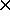MPHMXIMLM or increase the parameter MW in the FORTRAN source.
IRTN=1001 to 1004
Either one of MY,MPH,MXI,MLM is too large.
IRTN=1100
The enhancement function less than 1 at some y.

After initialization, an event can be generated by 1ex

```   CALL NLCPGN(PE1,WL,NL,HE1,HL,PD,DT,PMAX,IRR,NPH,PE2,HE2,PG,HG,
PROB,WGT,IRTN)```

Input variables

PE1
Array of dimension (0:3). Initial electron 4-momentum (eV/c).
WL
Laser photon energy (eV).
NL
Array of dimension 3. Unit vector along the laser direction.
HE1
Initial electron helicity. (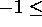HE1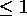).
HL
Laser helicity. (+1 or -1).
PD
Laser power density (Watt/m).
DT
Time interval times velocity of light. (meter).
PMAX
Maximum radiation probability in the given time interval. If the probability exceeds PMAX, return with IRTN=100 without event generation. You need to reduce the time interval DT.
If you set, for example, PMAX=0.1, then you are ignoring the probability (=PMAX=0.01) of two (and more) events within DT. A smaller PMAX is safer but more time consuming.
IRR
Random number seed.

Output variables

NPH
If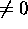, one event is created with NPH laser-photon absorption. If =0, no radiation (following variables are meaningless.)
PE2
Array of dimension (0:3). Final electron 4-momentum (eV/c).
HE2
Final electron helicity. (HE2).
PG
Array of dimension (0:3). Final photon 4-momentum (eV/c).
HG
Final photon helicity. (HG).
PROB
Calculated event probability. When an error occurs with IRTN=100, you have to multiply DT at least by a factor PROB/PMAX.
WGT
Weight factor of the event. It is always 1 if LENH=0 at the initialization. If not equal to 1, then you should asign a weight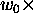WGT for the final photon end electron and let the initial electron survive with the weight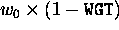, where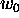is the weight of the initial electron before the event. If WGT=1, you should eliminate the initial electron and asign the weightfor the final photon and electron.
IRTN
Return code.

Possible errors

IRTN=1000
Initialization not yet done.
IRTN=1001is larger than XIMAX.
IRTN=1002is larger than LMMAX.
IRTN=100
The total rate exceeds PMAX.Next: Algorithm Up: Compton Process Previous: Formulas

Toshiaki Tauchi
Thu Dec 3 17:27:26 JST 1998